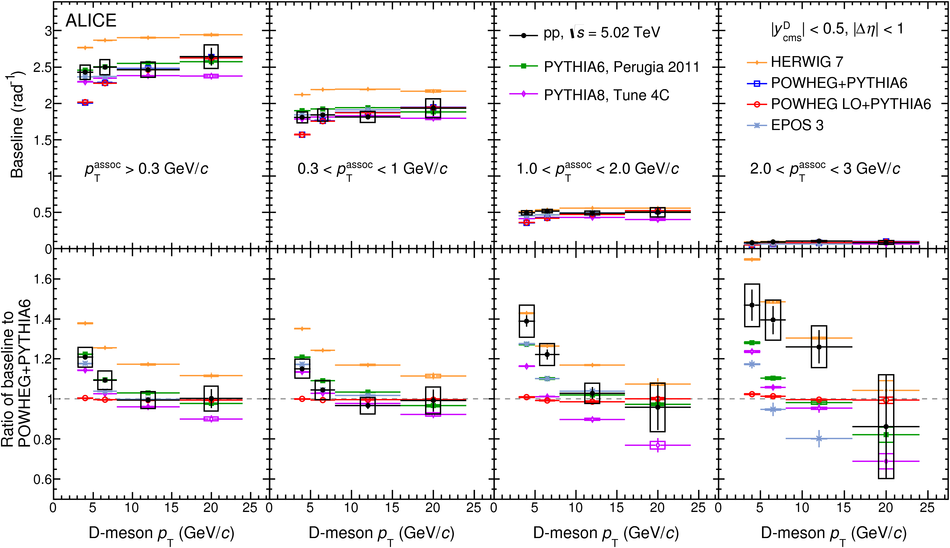# Azimuthal correlations of prompt D mesons with charged particles in pp and p-Pb collisions at $\sqrt{s_{\rm NN}}$ = 5.02 TeV

The measurement of the azimuthal-correlation function of prompt D mesons with charged particles in pp collisions at $\sqrt{s}$ = 5.02 TeV and p-Pb collisions at $\sqrt{s_{\rm NN}}$ = 5.02 TeV with the ALICE detector at the LHC is reported. The D$^{\rm 0}$, D$^{\rm +}$, and D$^{\rm *+}$ mesons, together with their charge conjugates, were reconstructed at midrapidity in the transverse momentum interval 3 <~ $p_{\rm T}$ <~ 24 GeV/c and correlated with charged particles having $p_{\rm T}$ > 0.3 GeV/c and pseudorapidity $|\eta| <~$ 0.8. The properties of the correlation peaks appearing in the near- and away-side regions (for $\Delta \varphi \approx$ 0 and $\Delta \varphi \approx \pi$, respectively) were extracted via a fit to the azimuthal correlation functions. The shape of the correlation functions and the near- and away-side peak features are found to be consistent in pp and p-Pb collisions, showing no modifications due to nuclear effects within uncertainties. The results are compared with predictions from Monte Carlo simulations performed with the PYTHIA, POWHEG+PYTHIA, HERWIG, and EPOS 3 event generators.

Submitted to: EPJC
e-Print: arXiv:1910.14403 | PDF | inSPIRE
CERN-EP-2019-239

Figures

## Figure 1

 Invariant mass (mass-difference) distributions of $\Dzero$, $\Dplus$ ($\Dstar$), and charge conjugates, candidates in three $\ptD$ intervals for pp collisions at $\s$ = 5.02 TeV (top row) and \pPb collisions at $\snn$ = 5.02 TeV (bottom row). The curves show the fit functions applied to the distributions. For the $\Dzero$, the dashed line represents the combinatorial background including the contribution of reflection candidates (see~). The values of the mean ($\mu$) and width ($\sigma$) of the signal peak, extracted from the fit, are also reported.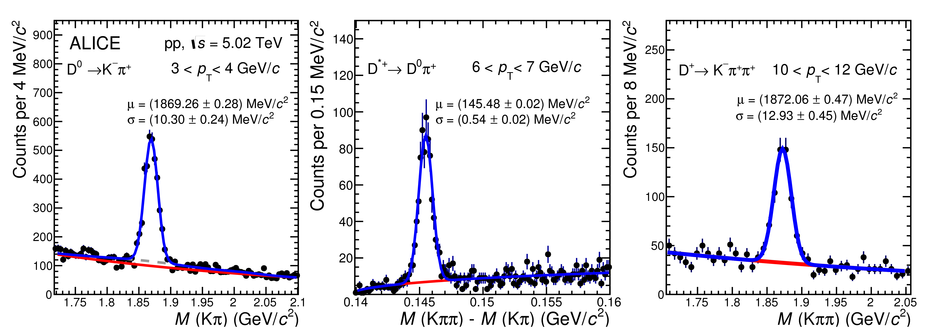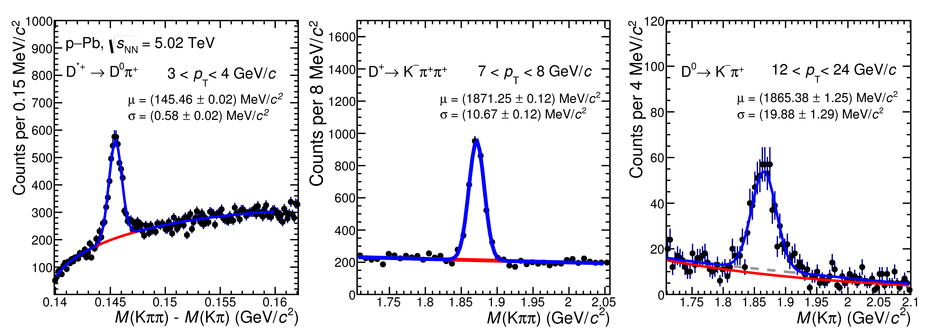## Figure 2

 Examples of the fit to the D-meson average azimuthal-correlation function, for $5 < \ptD < 8$~\GeVc, $\ptass > 0.3$~\GeVc in pp collisions (left), and for $8 < \ptD < 16$~\GeVc, $1 < \ptass < 2$~\GeVc in \pPb collisions (right). The statistical uncertainties are shown as vertical error bars. The fit function described in Eq.~\ref{equ:Fit} is shown as a red solid curve. Its different terms are shown separately: near-side generalised Gaussian function, away-side Gaussian function, and baseline constant term. The scale uncertainty (see Sec.~\ref{sec:Systematics}) is also reported for completeness.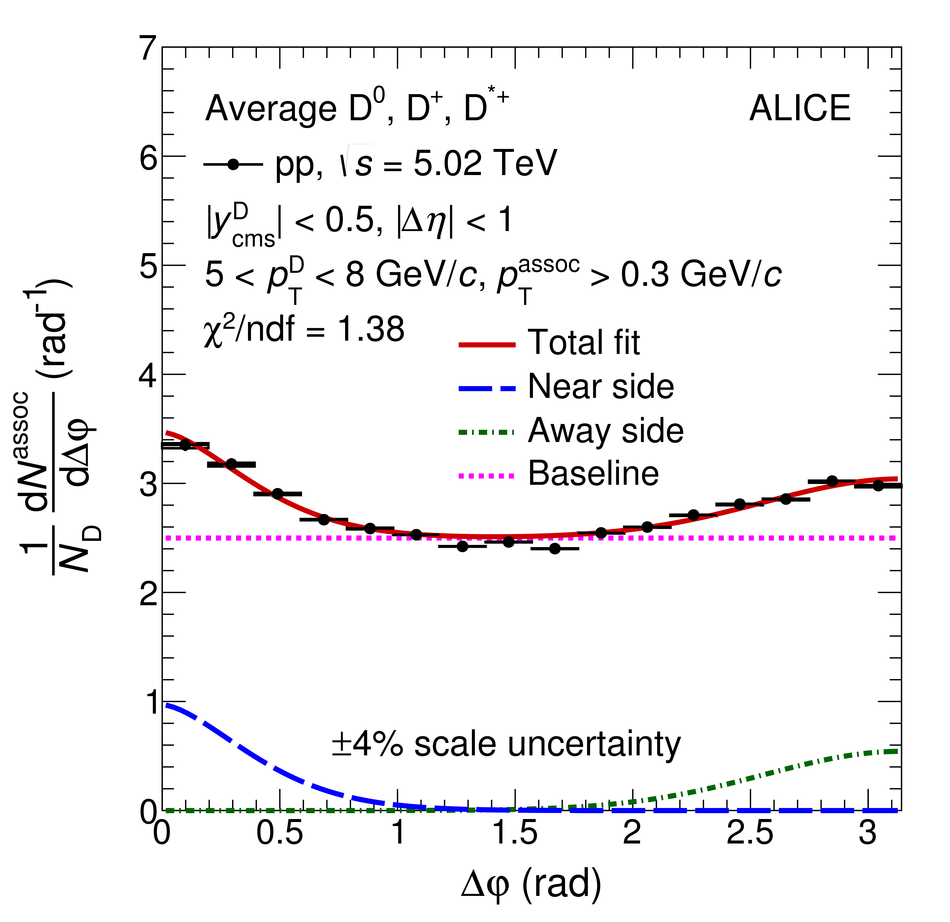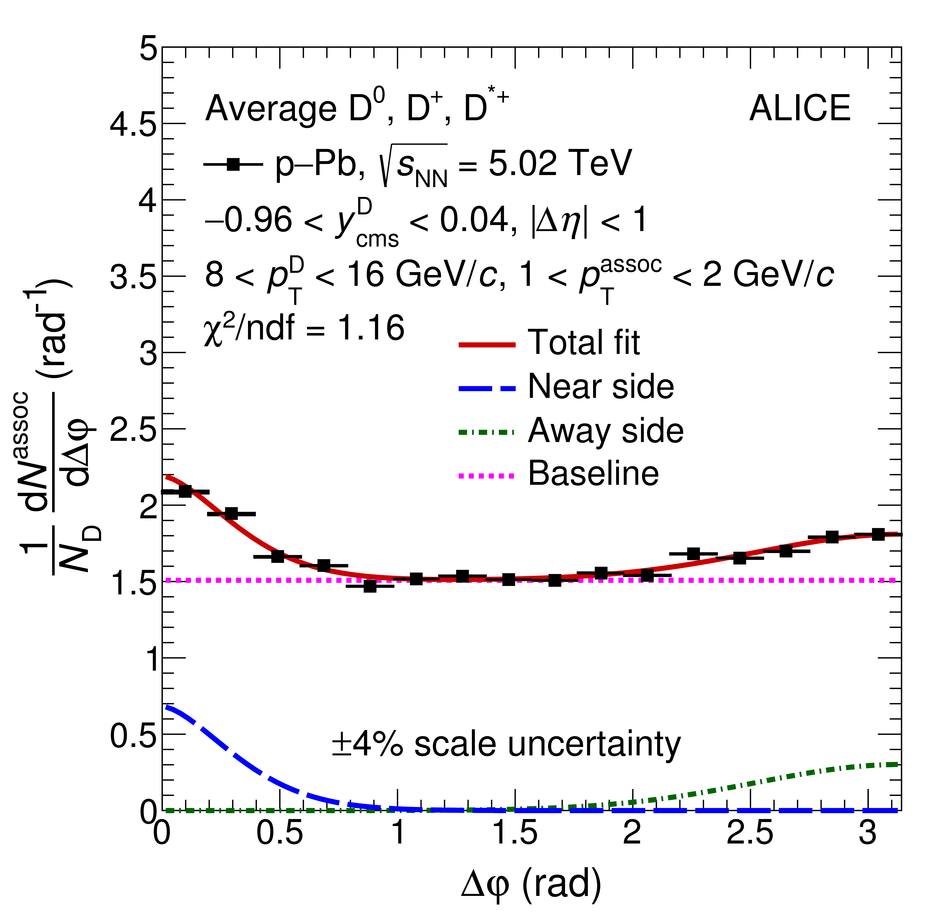## Figure 3

 Average of the azimuthal-correlation functions of $\Dzero$, $\Dplus$, and $\Dstar$ mesons with associated particles, after the subtraction of the baseline, in \pp collisions at ${\s = 5.02}$ \TeV and \pPb collisions at $\snn = 5.02$~\TeV, for $3 < \ptD < 5$~\GeVc, $5 < \ptD < 8$~\GeVc, $8 < \ptD < 16$~\GeVc, and $16 < \ptD < 24$~\GeVc (from left to right) and $\ptass > 0.3$~\GeVc, $0.3 < \ptass < 1$~\GeVc, $1 < \ptass < 2$~\GeVc, and $2 < \ptass < 3$~\GeVc (from top to bottom). Statistical and $\Dphi$-dependent systematic uncertainties are shown as vertical error bars and boxes, respectively, $\Dphi$-independent uncertainties are written as text. The uncertainties from the subtraction of the baseline are displayed as boxes at $\Dphi > \pi$.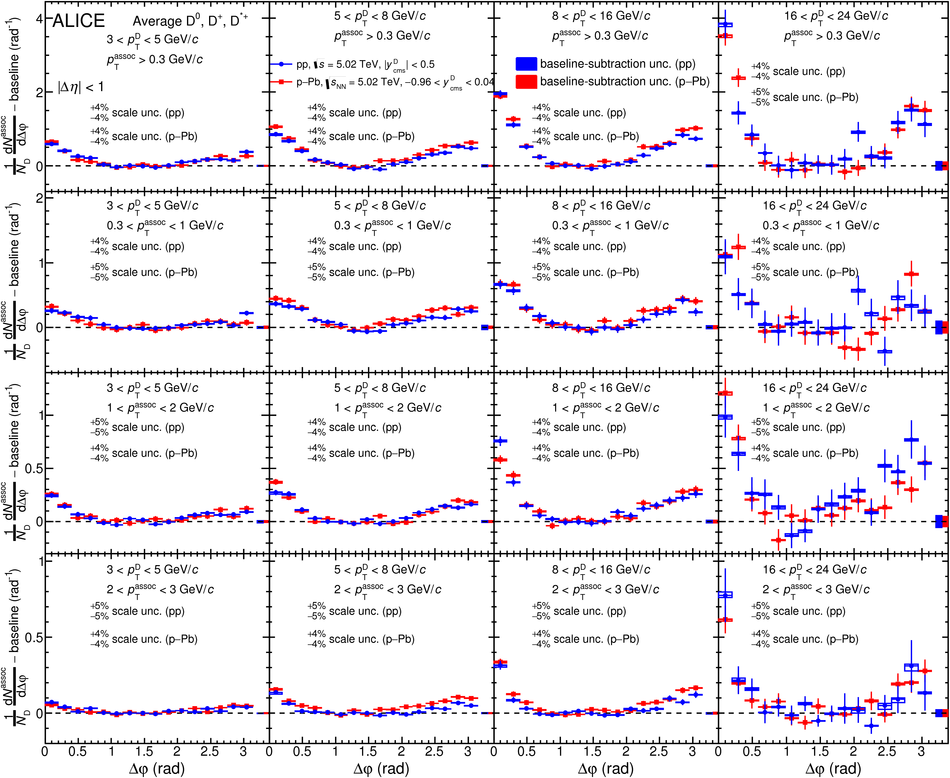## Figure 4

 Near-side peak associated yields (top row) and widths (bottom row) in \pp collisions at ${\s = 5.02}$ \TeV and \pPb collisions at ${\snn = 5.02}$ \TeV, as a function of the D-meson $\pt$, for ${\ptass > 0.3}$~\GeVc, ${0.3 < \ptass < 1}$~\GeVc, ${1 < \ptass < 2}$~\GeVc, and ${2 < \ptass < 3}$~\GeVc (from left to right). Statistical and systematic uncertainties are shown as vertical error bars and boxes, respectively.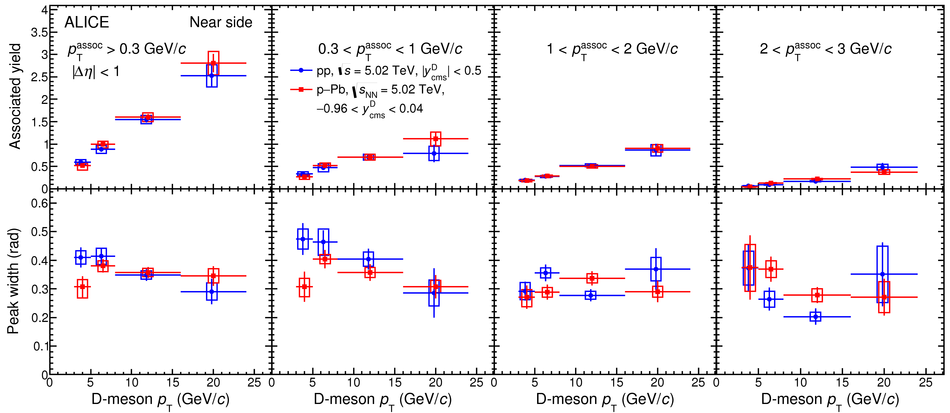## Figure 5

 Away-side peak associated yields (top row) and widths (bottom row) in \pp collisions at ${\s = 5.02}$ \TeV and \pPb collisions at ${\snn = 5.02}$ \TeV, as a function of the D-meson $\pt$, for ${\ptass > 0.3}$~\GeVc, ${0.3 < \ptass < 1}$~\GeVc, ${1 < \ptass < 2}$~\GeVc, and ${2 < \ptass < 3}$~\GeVc (from left to right). Statistical and systematic uncertainties are shown as vertical error bars and boxes, respectively.## Figure 6

 Average of the azimuthal-correlation functions of $\Dzero$, $\Dplus$, and $\Dstar$ mesons with associated particles, after the subtraction of the baseline, for \pPb collisions in three different centrality classes, 0--20\% (blue circles), 20--60\% (red squares), and 60--100\% (green diamonds). The functions are shown for $3 < \ptD < 5$~\GeVc, $5~< ~\ptD~< ~8$~\GeVc, and $8 < \ptD < 16$~\GeVc (from left to right) and $\ptass > 0.3$~\GeVc, $0.3 < \ptass < 1$~\GeVc, and $\ptass > 1$~\GeVc (from top to bottom). Statistical and $\Dphi$-dependent systematic uncertainties are shown as vertical error bars and boxes, respectively, while $\Dphi$-independent uncertainties are written as text. The uncertainties from the subtraction of the baseline are displayed as boxes at $\Dphi > \pi$.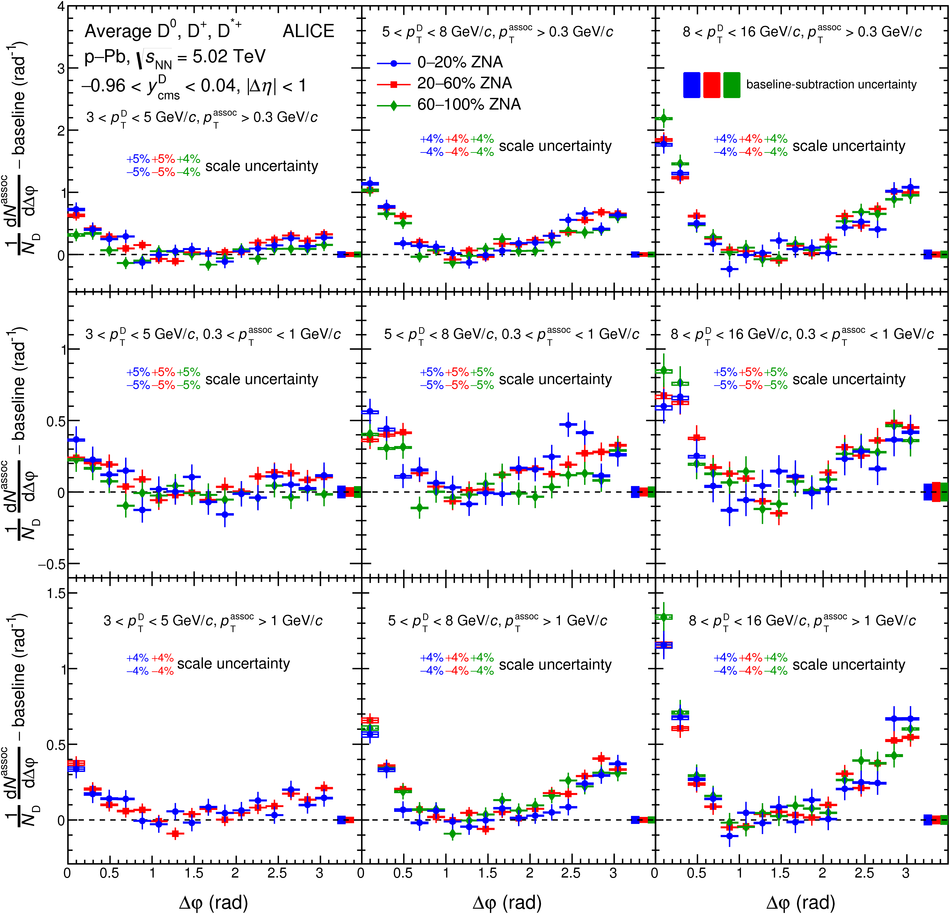## Figure 7

 Comparison of near-side associated peak yields (top row) and widths (bottom row) as a function of the D-meson $\pt$, for \pPb collisions in three different centrality classes, 0--20\% (blue circles), 20--60\% (red squares), and 60--100\% (green diamonds). The results are presented as a function of the D-meson $\pt$, for $\ptass > 0.3$~\GeVc, $0.3 < \ptass < 1$~\GeVc, and $\ptass > 1$~\GeVc (from left to right). Statistical and systematic uncertainties are shown as vertical error bars and boxes, respectively.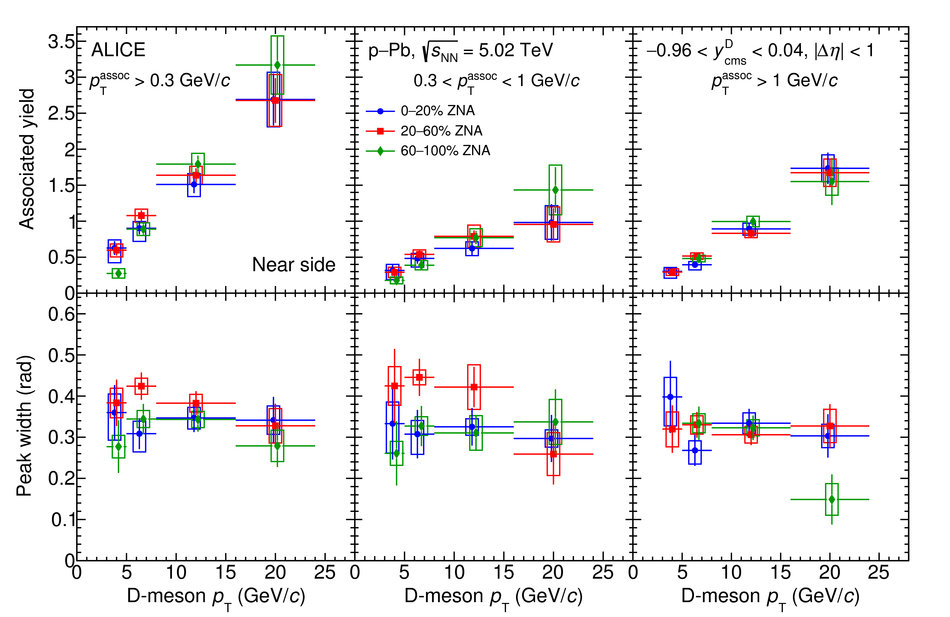## Figure 8

 The average of the azimuthal-correlation functions of $\Dzero$, $\Dplus$, and $\Dstar$ mesons with associated particles, after the subtraction of the baseline, in \pp collisions at $\s = 5.02$~\TeV, compared to predictions from the PYTHIA, POWHEG+PYTHIA6, POWHEG LO+PYTHIA6, HERWIG, and EPOS 3 event generators with various configurations (see text for details). The functions are shown for $3 < \ptD < 5$~\GeVc, $5 < \ptD < 8$~\GeVc, $8 < \ptD < 16$~\GeVc, and $16 < \ptD < 24$~\GeVc (from left to right) and $\ptass > 0.3$~\GeVc, $0.3 < \ptass < 1$~\GeVc, $1 < \ptass < 2$~\GeVc, and $2 < \ptass < 3$~\GeVc (from top to bottom). Statistical and $\Dphi$-dependent systematic uncertainties are shown as vertical error bars and boxes, respectively, while the $\Dphi$-independent uncertainties are written as text. The uncertainties from the subtraction of the baseline are displayed as boxes at $\Dphi > \pi$.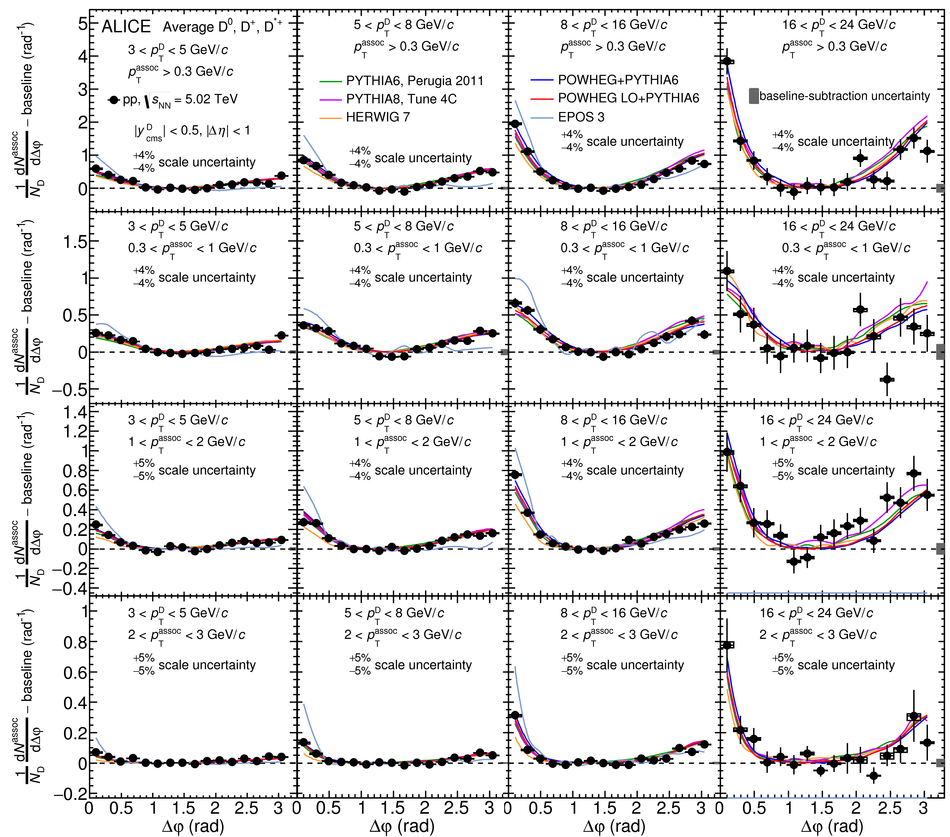## Figure 9

 Measurements of near-side associated peak yields (top row) and widths (third row down) in \pp collisions at $\s = 5.02$~\TeV, compared to predictions by the PYTHIA, POWHEG+PYTHIA6, POWHEG LO+PYTHIA6, HERWIG, and EPOS 3 event generators with various configurations (see text for details). The ratios of yield (width) values with respect to the predictions by POWHEG+PYTHIA6 are shown in the second (fourth) row down. Results are presented as a function of the D-meson $\pt$, for $\ptass > 0.3$~\GeVc, $0.3 < \ptass < 1$~\GeVc, $1 < \ptass < 2$~\GeVc, and $2 < \ptass < 3$~\GeVc (from left to right). Statistical and systematic uncertainties are shown as vertical error bars and boxes, respectively.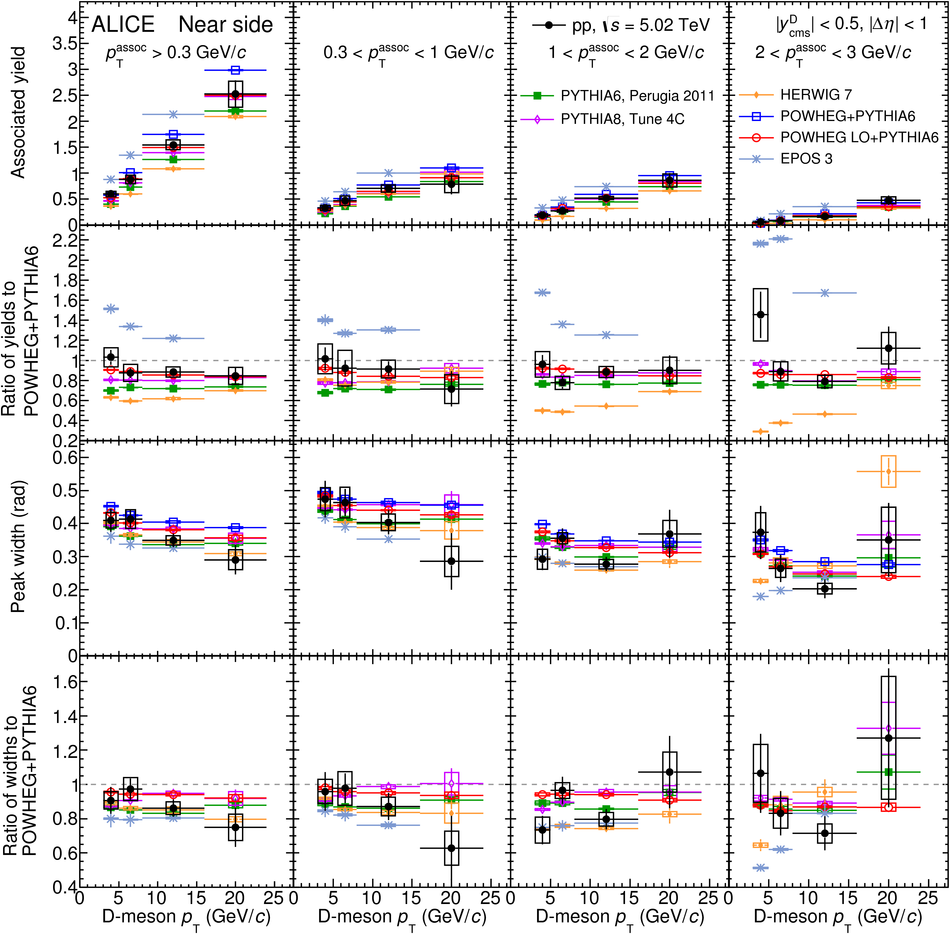## Figure 10

 Measurements of away-side associated peak yields (top row) and widths (third row down) in \pp collisions at $\s = 5.02$~\TeV, compared to predictions by the PYTHIA, POWHEG+PYTHIA6, POWHEG LO+PYTHIA6, and HERWIG event generators with various configurations (see text for details). The ratios of yield (width) values with respect to the predictions by POWHEG+PYTHIA6 are shown in the second (fourth) row down. Results are presented as a function of the D-meson $\pt$, for $\ptass > 0.3$~\GeVc, $0.3 < \ptass < 1$~\GeVc, $1 < \ptass < 2$~\GeVc, and $2 < \ptass < 3$~\GeVc (from left to right). Statistical and systematic uncertainties are shown as vertical error bars and boxes, respectively.## Figure 11

 Measurements of azimuthal-correlation function baseline height in \pp collisions at $\s = 5.02$ \TeV, compared to predictions by the PYTHIA, POWHEG+PYTHIA6, POWHEG LO+PYTHIA6, HERWIG, and EPOS 3 event generators with various configurations in the top row (see text for details). The ratios of baselines with respect to predictions by POWHEG+PYTHIA6 are shown in the bottom row. Results are presented as a function of the D-meson $\pt$, for $\ptass > 0.3$~\GeVc, $0.3 < \ptass < 1$~\GeVc, $1 < \ptass < 2$~\GeVc, and $2 < \ptass < 3$~\GeVc (from left to right). Statistical and systematic uncertainties are shown as vertical error bars and boxes, respectively.